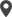# Odoo 16 Development Book

## Onchange with Compute Method

Odoo's most commonly used techniques for achieving some kind of business logic is Onchange and compute logic. A field that uses values from other fields to calculate its value is known as a computed field. This method is used to compute the value of a computed field and is generally referred to as compute methods; onchange methods are methods triggered by a change in the field value.

Let's look at both scenarios using an example. Consider developing a business model for managing rental orders. Duration and price for the unit duration are used for calculating the amount for the total rent. For this purpose, let us define as in the following code a monetary field total_rent, which is a computed field, meaning that its value is determined by using the values of either one or more other fields.

``````from odoo import models, fields
class VehicleRental(models.Model):
_name = "vehicle.rental"
_description = "Vehicle Rental"
hour_rate = fields.Monetary(string="Hour Rate",)
hours = fields.Integer(string="Hours")
total_rent = fields.Monetary(string='Total Rent',
compute='_compute_total_rent')
def _compute_total_rent(self):
for record in self:
record.total_rent = record.hour_rate*record.hours``````

The value of computed fields is usually dependent on other fields. Therefore, it is preferable to include the dependencies in the compute method. The ORM expects that the developer will declare these dependencies using the method's decorator depends(). When a change occurs in the dependent fields, ORM uses the provided dependencies to trigger the compute method.

``````from odoo import models, fields, api
class VehicleRental(models.Model):
_name = "vehicle.rental"
_description = "Vehicle Rental"
hour_rate = fields.Monetary(string="Hour Rate",)
hours = fields.Integer(string="Hours")
total_rent = fields.Monetary(string='Total Rent',
compute='_compute_total_rent')
@api.depends('hour_rate', 'hours')
def _compute_total_rent(self):
for record in self:
record.total_rent = record.hour_rate*record.hours``````

With Odoo's Onchange mechanism, a field's value can be changed or updated if any updates are made to the values of the other fields. Additionally, the onchange is only activated in the form view and is triggered whenever one of the specified fields is modified in that view. In the given example, the Reference for the rental order is achieved from a method. The onchange() decorator allows the feature to call the onchange method whenever the value of the form's vehicle_id field changes.

``````from odoo import models, fields, api
class VehicleRental(models.Model):
_name = "vehicle.rental"
_description = "Vehicle Rental"
name = fields.Char(string="Ref.")
vehicle_id = fields.Many2one('fleet.vehicle', string="Vehicle")
@api.onchange('vehicle_id')
def _onchange_vehicle(self):
self.name = "Rental Order for %s" % self.vehicle_id.name
``````Calicut

Cybrosys Technologies Pvt. Ltd.
Neospace, Kinfra Techno Park
Kakkancherry, Calicut
Kerala, India - 673635London

Cybrosys Limited
Alpha House,
100 Borough High Street, London,
SE1 1LB, United KingdomKochi

Cybrosys Technologies Pvt. Ltd.
1st Floor, Thapasya Building,
Kochi, India - 682030.Bangalore

Cybrosys Techno Solutions
The Estate, 8th Floor,